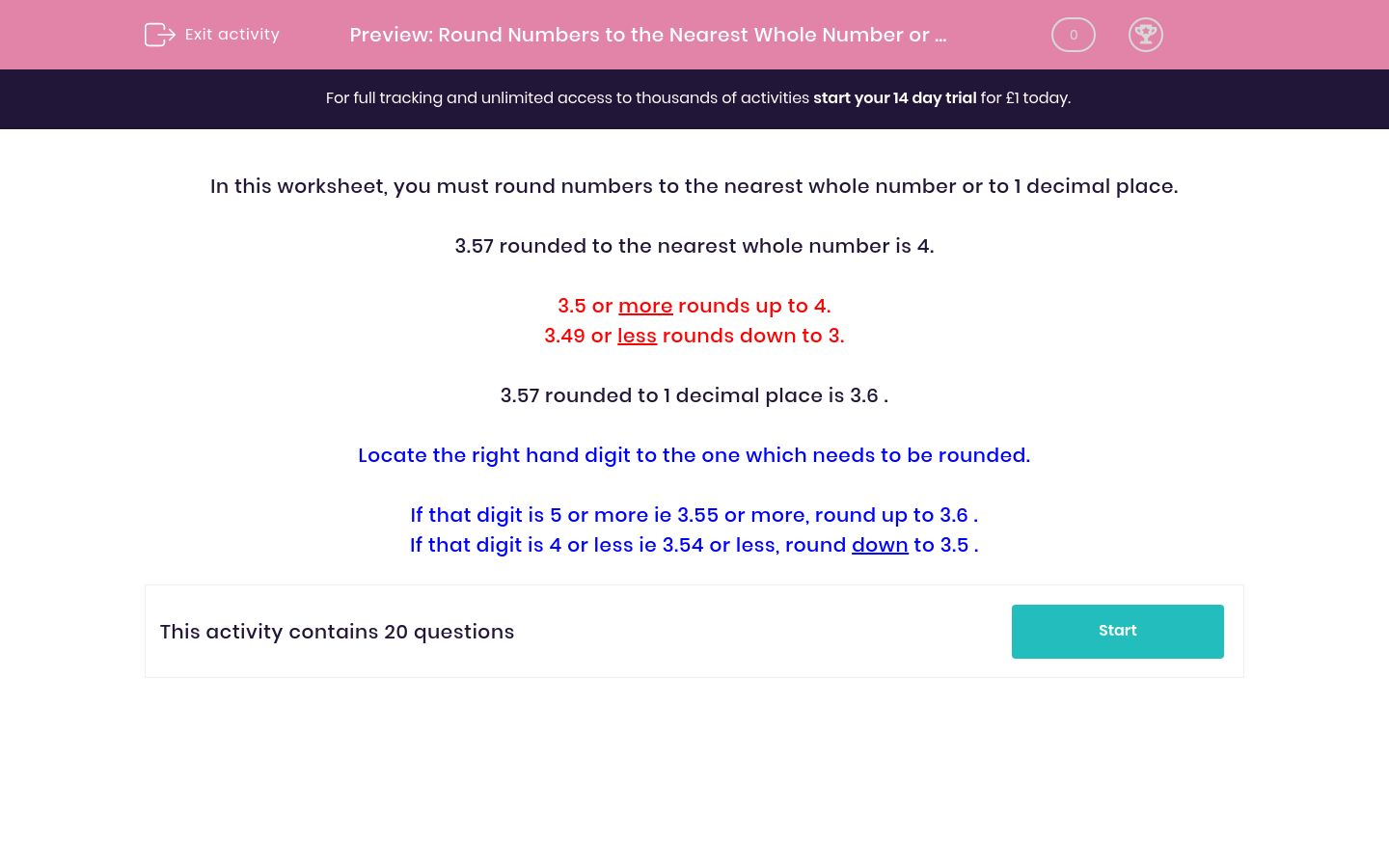# Round Numbers to the Nearest Whole Number or to 1 Decimal Place

In this worksheet, students revise and practise rounding numbers to the nearest whole number or to 1 decimal place.Key stage:  KS 2

Curriculum topic:   Maths and Numerical Reasoning

Curriculum subtopic:   Place Value

Difficulty level:### QUESTION 1 of 10

In this worksheet, you must round numbers to the nearest whole number or to 1 decimal place.

3.57 rounded to the nearest whole number is 4.

3.5 or more rounds up to 4.
3.49 or less rounds down to 3.

3.57 rounded to 1 decimal place is 3.6 .

Locate the right hand digit to the one which needs to be rounded.

If that digit is 5 or more ie 3.55 or more, round up to 3.6 .
If that digit is 4 or less ie 3.54 or less, round down to 3.5 .

Round the following number to the nearest whole number.

8.72 =

Round the following number to the nearest whole number.

1.76 =

Round the following number to the nearest whole number.

1.96 =

Round the following number to the nearest whole number.

5.49 =

Round the following number to the nearest whole number.

4.15 =

Round the following number to the nearest whole number.

3.21 =

Round the following number to the nearest whole number.

8.61 =

Round the following number to the nearest whole number.

7.74 =

Round the following number to the nearest whole number.

6.99 =

Round the following number to the nearest whole number.

4.09 =

Round the following number to 1 decimal place.

4.09 =

Round the following number to 1 decimal place.

4.67 =

Round the following number to 1 decimal place.

4.64 =

Round the following number to 1 decimal place.

2.92 =

Round the following number to 1 decimal place.

2.97 =

Round the following number to the nearest whole number.

2.97 =

Round the following number to 1 decimal place.

8.49 =

Round the following number to the nearest whole number.

8.49 =

Round the following number to 1 decimal place.

8.99 =

Round the following number to 1 decimal place.

8.03 =
• Question 1

Round the following number to the nearest whole number.

8.72 =
9
• Question 2

Round the following number to the nearest whole number.

1.76 =
2
• Question 3

Round the following number to the nearest whole number.

1.96 =
2
• Question 4

Round the following number to the nearest whole number.

5.49 =
5
• Question 5

Round the following number to the nearest whole number.

4.15 =
4
• Question 6

Round the following number to the nearest whole number.

3.21 =
3
• Question 7

Round the following number to the nearest whole number.

8.61 =
9
• Question 8

Round the following number to the nearest whole number.

7.74 =
8
• Question 9

Round the following number to the nearest whole number.

6.99 =
7
• Question 10

Round the following number to the nearest whole number.

4.09 =
4
• Question 11

Round the following number to 1 decimal place.

4.09 =
4.1
• Question 12

Round the following number to 1 decimal place.

4.67 =
4.7
• Question 13

Round the following number to 1 decimal place.

4.64 =
4.6
• Question 14

Round the following number to 1 decimal place.

2.92 =
2.9
• Question 15

Round the following number to 1 decimal place.

2.97 =
3.0
• Question 16

Round the following number to the nearest whole number.

2.97 =
3
EDDIE SAYS
3 to the nearest whole number 3.0 to 1 decimal place
• Question 17

Round the following number to 1 decimal place.

8.49 =
8.5
• Question 18

Round the following number to the nearest whole number.

8.49 =
8
EDDIE SAYS
8 to the nearest whole number 8.5 to 1 decimal place
• Question 19

Round the following number to 1 decimal place.

8.99 =
9.0
• Question 20

Round the following number to 1 decimal place.

8.03 =
8.0
---- OR ----

Sign up for a £1 trial so you can track and measure your child's progress on this activity.

### What is EdPlace?

We're your National Curriculum aligned online education content provider helping each child succeed in English, maths and science from year 1 to GCSE. With an EdPlace account you’ll be able to track and measure progress, helping each child achieve their best. We build confidence and attainment by personalising each child’s learning at a level that suits them.

Get started